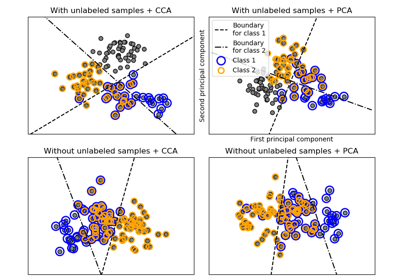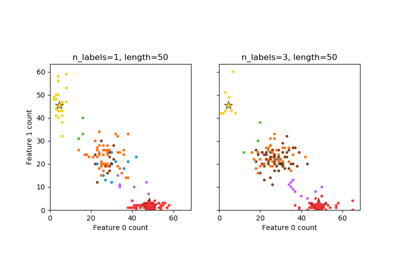/scikit-learn

# sklearn.datasets.make_multilabel_classification

`sklearn.datasets.make_multilabel_classification(n_samples=100, n_features=20, n_classes=5, n_labels=2, length=50, allow_unlabeled=True, sparse=False, return_indicator=’dense’, return_distributions=False, random_state=None)` [source]

Generate a random multilabel classification problem.

For each sample, the generative process is:
• pick the number of labels: n ~ Poisson(n_labels)
• n times, choose a class c: c ~ Multinomial(theta)
• pick the document length: k ~ Poisson(length)
• k times, choose a word: w ~ Multinomial(theta_c)

In the above process, rejection sampling is used to make sure that n is never zero or more than `n_classes`, and that the document length is never zero. Likewise, we reject classes which have already been chosen.

Read more in the User Guide.

Parameters: `n_samples : int, optional (default=100)` The number of samples. `n_features : int, optional (default=20)` The total number of features. `n_classes : int, optional (default=5)` The number of classes of the classification problem. `n_labels : int, optional (default=2)` The average number of labels per instance. More precisely, the number of labels per sample is drawn from a Poisson distribution with `n_labels` as its expected value, but samples are bounded (using rejection sampling) by `n_classes`, and must be nonzero if `allow_unlabeled` is False. `length : int, optional (default=50)` The sum of the features (number of words if documents) is drawn from a Poisson distribution with this expected value. `allow_unlabeled : bool, optional (default=True)` If `True`, some instances might not belong to any class. `sparse : bool, optional (default=False)` If `True`, return a sparse feature matrix New in version 0.17: parameter to allow sparse output. `return_indicator : ‘dense’ (default) | ‘sparse’ | False` If `dense` return `Y` in the dense binary indicator format. If `'sparse'` return `Y` in the sparse binary indicator format. `False` returns a list of lists of labels. `return_distributions : bool, optional (default=False)` If `True`, return the prior class probability and conditional probabilities of features given classes, from which the data was drawn. `random_state : int, RandomState instance or None (default)` Determines random number generation for dataset creation. Pass an int for reproducible output across multiple function calls. See Glossary. `X : array of shape [n_samples, n_features]` The generated samples. `Y : array or sparse CSR matrix of shape [n_samples, n_classes]` The label sets. `p_c : array, shape [n_classes]` The probability of each class being drawn. Only returned if `return_distributions=True`. `p_w_c : array, shape [n_features, n_classes]` The probability of each feature being drawn given each class. Only returned if `return_distributions=True`.

## Examples using `sklearn.datasets.make_multilabel_classification`Multilabel classificationPlot randomly generated multilabel dataset